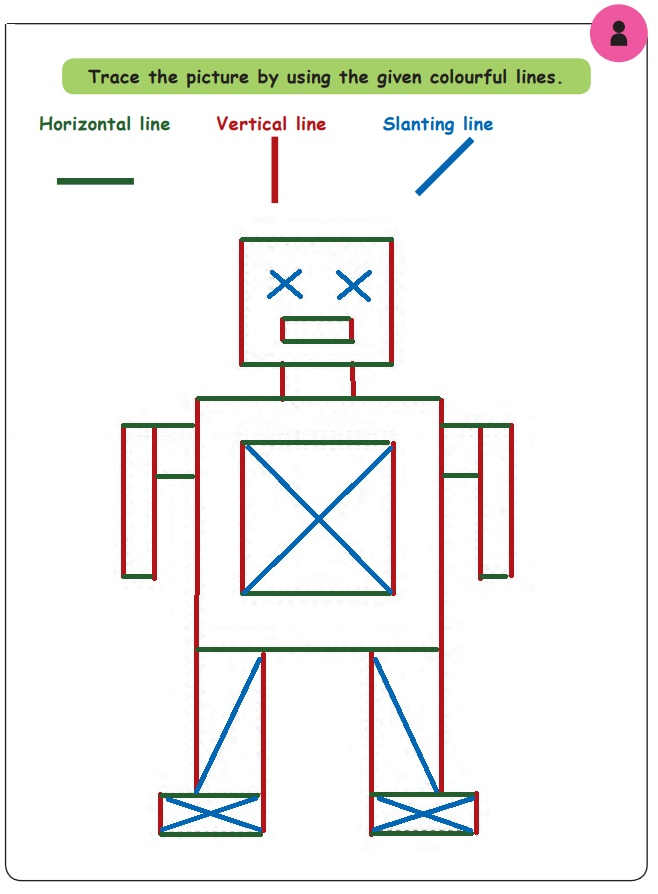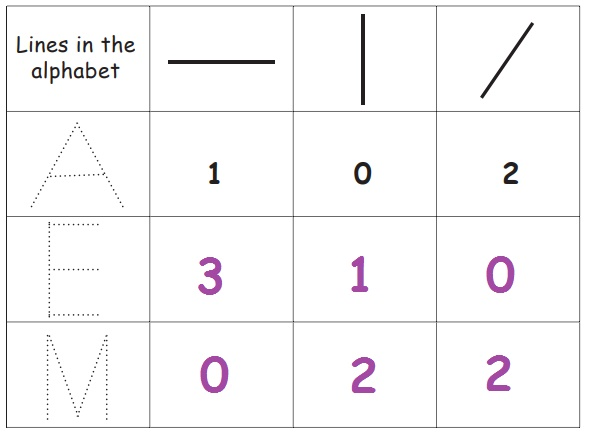Home | | Maths 1st Std | Introduction to straight lines

# Introduction to straight lines

Keywords: Horizantal line, Vertical line, Slanting line

Introduction to straight lines

Keywords: Horizantal line, Vertical line, Slanting line

Learn

Observe and trace the lines.

Horizontal line, Vertical line, Slanting linePractice

Trace the picture by using the given colourful lines.Teacher's Note: Teacher needs to make the children familiarize with the variety of slanting lines.

Think like a Mathematician

Trace and observe the type of lines in the alphabet. Count and write.Activity

Draw the pictures of your own using Horizontal line, Vertical line and Slanting line. One is done for you.Tags : Geometry | Term 2 Chapter 1 | 1st Maths , 1st Maths : Term 2 Unit 1 : Geometry
Study Material, Lecturing Notes, Assignment, Reference, Wiki description explanation, brief detail
1st Maths : Term 2 Unit 1 : Geometry : Introduction to straight lines | Geometry | Term 2 Chapter 1 | 1st Maths